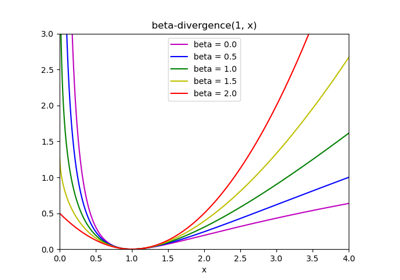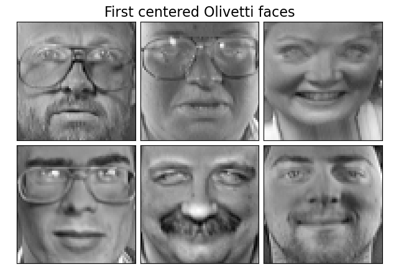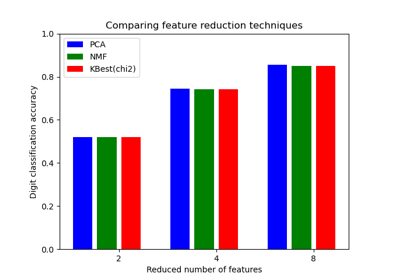sklearn.decomposition.NMF¶

class sklearn.decomposition.NMF(n_components=None, *, init=None, solver='cd', beta_loss='frobenius', tol=0.0001, max_iter=200, random_state=None, alpha=0.0, l1_ratio=0.0, verbose=0, shuffle=False)[source]

Non-Negative Matrix Factorization (NMF)

Find two non-negative matrices (W, H) whose product approximates the non- negative matrix X. This factorization can be used for example for dimensionality reduction, source separation or topic extraction.

The objective function is:

0.5 * ||X - WH||_Fro^2
+ alpha * l1_ratio * ||vec(W)||_1
+ alpha * l1_ratio * ||vec(H)||_1
+ 0.5 * alpha * (1 - l1_ratio) * ||W||_Fro^2
+ 0.5 * alpha * (1 - l1_ratio) * ||H||_Fro^2

Where:

||A||_Fro^2 = \sum_{i,j} A_{ij}^2 (Frobenius norm)
||vec(A)||_1 = \sum_{i,j} abs(A_{ij}) (Elementwise L1 norm)

For multiplicative-update (‘mu’) solver, the Frobenius norm (0.5 * ||X - WH||_Fro^2) can be changed into another beta-divergence loss, by changing the beta_loss parameter.

The objective function is minimized with an alternating minimization of W and H.

Read more in the User Guide.

Parameters
n_componentsint or None

Number of components, if n_components is not set all features are kept.

initNone | ‘random’ | ‘nndsvd’ | ‘nndsvda’ | ‘nndsvdar’ | ‘custom’

Method used to initialize the procedure. Default: None. Valid options:

• None: ‘nndsvd’ if n_components <= min(n_samples, n_features),

otherwise random.

• ‘random’: non-negative random matrices, scaled with:

sqrt(X.mean() / n_components)

• ‘nndsvd’: Nonnegative Double Singular Value Decomposition (NNDSVD)

initialization (better for sparseness)

• ‘nndsvda’: NNDSVD with zeros filled with the average of X

(better when sparsity is not desired)

• ‘nndsvdar’: NNDSVD with zeros filled with small random values

(generally faster, less accurate alternative to NNDSVDa for when sparsity is not desired)

• ‘custom’: use custom matrices W and H

solver‘cd’ | ‘mu’

Numerical solver to use: ‘cd’ is a Coordinate Descent solver. ‘mu’ is a Multiplicative Update solver.

New in version 0.17: Coordinate Descent solver.

New in version 0.19: Multiplicative Update solver.

beta_lossfloat or string, default ‘frobenius’

String must be in {‘frobenius’, ‘kullback-leibler’, ‘itakura-saito’}. Beta divergence to be minimized, measuring the distance between X and the dot product WH. Note that values different from ‘frobenius’ (or 2) and ‘kullback-leibler’ (or 1) lead to significantly slower fits. Note that for beta_loss <= 0 (or ‘itakura-saito’), the input matrix X cannot contain zeros. Used only in ‘mu’ solver.

New in version 0.19.

tolfloat, default: 1e-4

Tolerance of the stopping condition.

max_iterinteger, default: 200

Maximum number of iterations before timing out.

random_stateint, RandomState instance, default=None

Used for initialisation (when init == ‘nndsvdar’ or ‘random’), and in Coordinate Descent. Pass an int for reproducible results across multiple function calls. See Glossary.

Constant that multiplies the regularization terms. Set it to zero to have no regularization.

New in version 0.17: alpha used in the Coordinate Descent solver.

l1_ratiodouble, default: 0.

The regularization mixing parameter, with 0 <= l1_ratio <= 1. For l1_ratio = 0 the penalty is an elementwise L2 penalty (aka Frobenius Norm). For l1_ratio = 1 it is an elementwise L1 penalty. For 0 < l1_ratio < 1, the penalty is a combination of L1 and L2.

New in version 0.17: Regularization parameter l1_ratio used in the Coordinate Descent solver.

verbosebool, default=False

Whether to be verbose.

shuffleboolean, default: False

If true, randomize the order of coordinates in the CD solver.

New in version 0.17: shuffle parameter used in the Coordinate Descent solver.

Attributes
components_array, [n_components, n_features]

Factorization matrix, sometimes called ‘dictionary’.

n_components_integer

The number of components. It is same as the n_components parameter if it was given. Otherwise, it will be same as the number of features.

reconstruction_err_number

Frobenius norm of the matrix difference, or beta-divergence, between the training data X and the reconstructed data WH from the fitted model.

n_iter_int

Actual number of iterations.

References

Cichocki, Andrzej, and P. H. A. N. Anh-Huy. “Fast local algorithms for large scale nonnegative matrix and tensor factorizations.” IEICE transactions on fundamentals of electronics, communications and computer sciences 92.3: 708-721, 2009.

Fevotte, C., & Idier, J. (2011). Algorithms for nonnegative matrix factorization with the beta-divergence. Neural Computation, 23(9).

Examples

>>> import numpy as np
>>> X = np.array([[1, 1], [2, 1], [3, 1.2], [4, 1], [5, 0.8], [6, 1]])
>>> from sklearn.decomposition import NMF
>>> model = NMF(n_components=2, init='random', random_state=0)
>>> W = model.fit_transform(X)
>>> H = model.components_

Methods

 fit(X[, y]) Learn a NMF model for the data X. fit_transform(X[, y, W, H]) Learn a NMF model for the data X and returns the transformed data. get_params([deep]) Get parameters for this estimator. Transform data back to its original space. set_params(**params) Set the parameters of this estimator. transform(X) Transform the data X according to the fitted NMF model
__init__(n_components=None, *, init=None, solver='cd', beta_loss='frobenius', tol=0.0001, max_iter=200, random_state=None, alpha=0.0, l1_ratio=0.0, verbose=0, shuffle=False)[source]

Initialize self. See help(type(self)) for accurate signature.

fit(X, y=None, **params)[source]

Learn a NMF model for the data X.

Parameters
X{array-like, sparse matrix}, shape (n_samples, n_features)

Data matrix to be decomposed

yIgnored
Returns
self
fit_transform(X, y=None, W=None, H=None)[source]

Learn a NMF model for the data X and returns the transformed data.

This is more efficient than calling fit followed by transform.

Parameters
X{array-like, sparse matrix}, shape (n_samples, n_features)

Data matrix to be decomposed

yIgnored
Warray-like, shape (n_samples, n_components)

If init=’custom’, it is used as initial guess for the solution.

Harray-like, shape (n_components, n_features)

If init=’custom’, it is used as initial guess for the solution.

Returns
Warray, shape (n_samples, n_components)

Transformed data.

get_params(deep=True)[source]

Get parameters for this estimator.

Parameters
deepbool, default=True

If True, will return the parameters for this estimator and contained subobjects that are estimators.

Returns
paramsmapping of string to any

Parameter names mapped to their values.

inverse_transform(W)[source]

Transform data back to its original space.

Parameters
W{array-like, sparse matrix}, shape (n_samples, n_components)

Transformed data matrix

Returns
X{array-like, sparse matrix}, shape (n_samples, n_features)

Data matrix of original shape

New in version 0.18: ..

set_params(**params)[source]

Set the parameters of this estimator.

The method works on simple estimators as well as on nested objects (such as pipelines). The latter have parameters of the form <component>__<parameter> so that it’s possible to update each component of a nested object.

Parameters
**paramsdict

Estimator parameters.

Returns
selfobject

Estimator instance.

transform(X)[source]

Transform the data X according to the fitted NMF model

Parameters
X{array-like, sparse matrix}, shape (n_samples, n_features)

Data matrix to be transformed by the model

Returns
Warray, shape (n_samples, n_components)

Transformed data

Examples using sklearn.decomposition.NMF¶